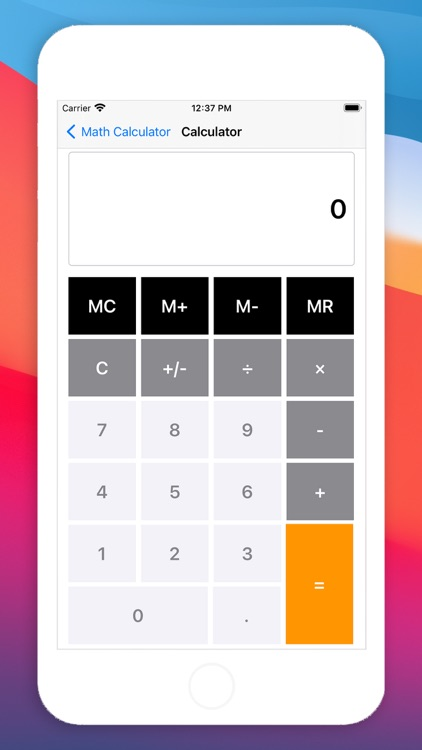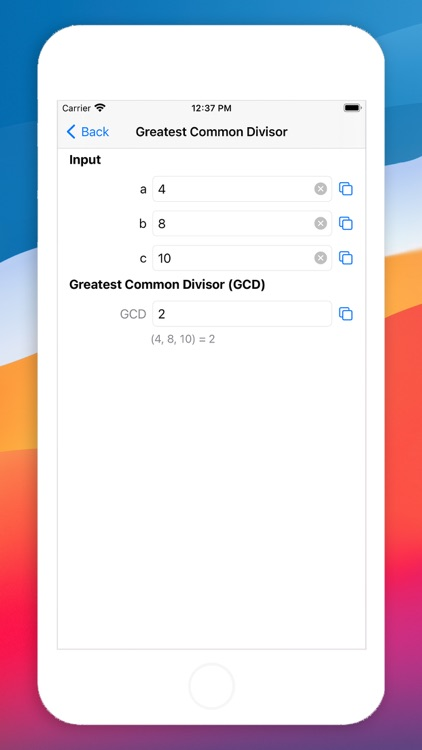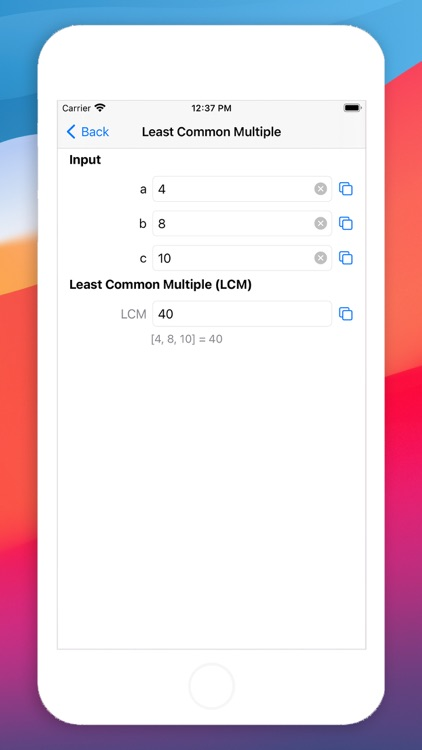## Math Calculator supports the calculation of common mathematical formulas: Hex Converter, Greatest Common Divisor, Least Common Multiple, Prime Factorization, Rooting, Exponentiation, Trigonometric Function, Inverse Trigonometric Function, Hyperbolic Function, Logarithm, Angular Transformation# Math Calculator - No Ads

by Xi An Yixueyiyong Software Technology Co., Ltd.Math Calculator supports the calculation of common mathematical formulas: Hex Converter, Greatest Common Divisor, Least Common Multiple, Prime Factorization, Rooting, Exponentiation, Trigonometric Function, Inverse Trigonometric Function, Hyperbolic Function, Logarithm, Angular Transformation.### App Details

Version
22.1
Rating
(2)
Size
0Mb
Genre
Utilities Reference
Last updated
August 20, 2021
Release date
June 11, 2015

• \$0.99

### App Screenshots### App Store Description

Math Calculator supports the calculation of common mathematical formulas: Hex Converter, Greatest Common Divisor, Least Common Multiple, Prime Factorization, Rooting, Exponentiation, Trigonometric Function, Inverse Trigonometric Function, Hyperbolic Function, Logarithm, Angular Transformation.

* Compatibility